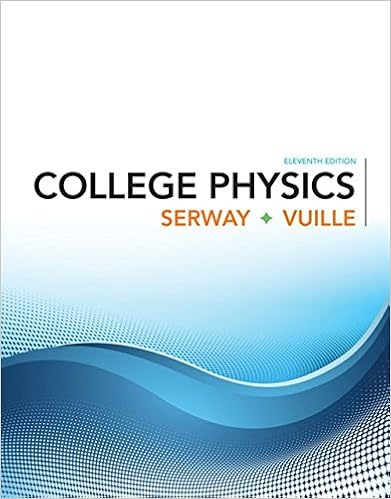# 44 weight the magnitude of the gravitational force

• 131

This preview shows page 28 - 53 out of 131 pages.

##### We have textbook solutions for you!
The document you are viewing contains questions related to this textbook.The document you are viewing contains questions related to this textbook.
Chapter 4 / Exercise 37
College Physics
Serway/VuilleExpert Verified
4.4 WeightThe magnitude of the gravitational force acting on an object of mass mnear the Earth’s surface is called the weight wof the objectw = m gis a special case of Newton’s Second Lawgis the acceleration due to gravityg can also be found from the Law of Universal Gravitation
##### We have textbook solutions for you!
The document you are viewing contains questions related to this textbook.The document you are viewing contains questions related to this textbook.
Chapter 4 / Exercise 37
College Physics
Serway/VuilleExpert Verified
More about weightWeight is notan inherent property of an object Mass isan inherent propertyWeight depends upon location
30Newton’s LawsDemonstration:Scales actually measure the Normal force, not the gravitational (weight) force, so their readings can fluctuate and change depending on circumstances.Scales in an elevator.
31Newton’s LawsWhen the elevator is at rest:a=0N=mgScale Reading=Weight
32Newton’s LawsWhen the elevator is accelerating upward:a>0N>mgScale Reading>Weight
33Newton’s LawsWhen the elevator is traveling at constant speed:a=0N=mgScale Reading=Weight
34Newton’s LawsWhen the elevator is decelerating and coming to rest:a<0N<mgScale Reading<Weight
35Now its your turn!What will happen to the scale reading as the elevator goes back down to the 1stfloor?What will happen if the elevator encounters a free fall?
An object can move even when no force acts on it.
If an object isn't moving, no external forces act on it.
If a single force acts on an object, the object accelerates.
If an object accelerates, a force is acting on it.1.True2.False
If an object isn’t accelerating, no external force is acting on it.
If the net force acting on an object is in the positive x-direction, the object moves only in the positive x-direction.
Which has a greater value, a newton of gold won on Earth or a newton of gold won on the Moon?Exactly …what is Force?
4.5 Newton’s Third LawIf object 1 and object 2 interact, the force exerted by object 1 on object 2 is equal in magnitude but opposite in direction to the force exerted by object 2 on object 1.Equivalent to saying a single isolated force cannot exist1221FFrr
•••